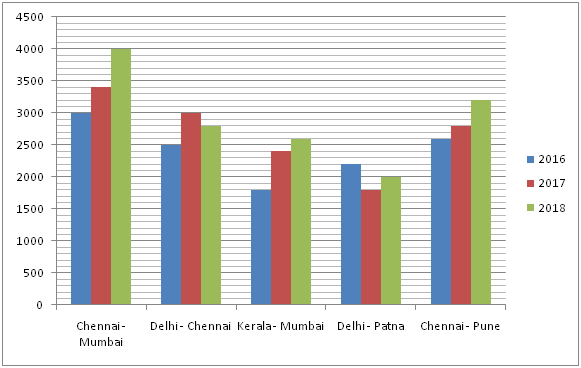# CWC/FCI Prelims 2019 – Quantitative Aptitude Questions (Day-09)

Dear Readers, Exam Race for the Year 2019 has already started, To enrich your preparation here we are providing new series of Practice Questions on Quantitative Aptitude – Section for CWC/FCI Exam. Aspirants, practice these questions on a regular basis to improve your score in aptitude section. Start your effective preparation from the right beginning to get success in upcoming CWC/FCI Exam.

[WpProQuiz 5478]

Directions (Q. 1 – 5): What value should come in place of question mark (?) in the following questions?

1) √16384 ÷ 4 × 16 =? – 18312 ÷ 12 + 142

a) 1678

b) 1842

c) 1426

d) 2154

e) None of these

2) (7/9) of 4068 + 77 % of 1200 = (?)2 – (128 ÷ 16)

a) 64

b) 62

c) 58

d) 56

e) None of these

3) (8 × 8)5 ÷ (512 ÷ 8)2 × (8 × 64)3 = (8)? + 5

a) 12

b) 8

c) 6

d) 10

e) None of these

4) ? % of (75 × 4 – 150) = 420 + 752

a) 4280

b) 4520

c) 4030

d) 5060

e) None of these

5) (7/84) of (12/156) of 4680 = 45 – ?

a) 15

b) 20

c) 25

d) 30

e) None of these

Directions (Q. 6 – 10): Study the following information carefully and answer the given questions:

The following bar graph shows the total train fare of 5 different routes in 3 different years.6) Find the difference between the total train fare in the year 2016 to that of 2018 of all the given routes together?

a) 2500

b) 2800

c) 3200

d) 3500

e) None of these

7) Find the ratio between the total train fares of Delhi – Chennai to that of Chennai – Pune in all the given years together?

a) 67: 72

b) 125: 133

c) 219: 221

d) 83: 86

e) None of these

8) Total train fares in the year 2017 for the routes of Chennai – Mumbai, Kerala – Mumbai and Chennai – Pune together is approximately what percentage of total train fares in the year 2016 for the routes of Delhi – Chennai, Kerala – Mumbai and Delhi – Patna together?

a) 145 %

b) 132 %

c) 123 %

d) 106 %

e) 92 %

9) Find the average train fare in the year 2017 of all the given years together?

a) 2240

b) 2820

c) 3060

d) 2680

e) None of these

10) Total train fare in the year 2018 of all the given routes together is approximately what percentage more/less than the total train fare in the year 2017 of all the given routes together?

a) 15 % more

b) 9 % more

c) 20 % less

d) 9 % less

e) 20 % more

Direction (1-5) :

√16384 ÷ 4 × 16 = x – 18312 ÷ 12 + 142

(128/4)*16 = x – (18312/12) + 196

512 = x – 1526 + 196

X = 512 + 1526 – 196 = 1842

(7/9)*4068 + (77/100)*1200 = x2 – (128/16)

3164 + 924 + 8 = x2

X2 = 4096

X = 64

(82)5 ÷ (512/8)2 × (8 × 82)3 = (8)x + 5

810 ÷ (64)2 × (83)3 = (8)x + 5

810 ÷ 84 × 89 = (8)x + 5

810 – 4 + 9 = 8x + 5

815 = 8x + 5

15 = x + 5

X = 10

x % of (75 × 4 – 150) = 420 + 752

(x/100)*(300 – 150) = 420 + 5625

(x/100)*150 = 6045

3x/2 = 6045

X = 6045*(2/3) = 4030

(7/84)*(12/156)*4680 = 45 – x

30 = 45 – x

X = 45 – 30 = 15

Direction (1-5) :

The total train fare in the year 2016 of all the given routes together

= > 3000 + 2500 + 1800 + 2200 + 2600 = 12100

The total train fare in the year 2018 of all the given routes together

= > 4000 + 2800 + 2600 + 2000 + 3200 = 14600

Required difference = 14600 – 12100 = 2500

The total train fares of Delhi – Chennai in all the given years together

= > 2500 + 3000 + 2800 = 8300

The total train fares of Chennai – Pune in all the given years together

= > 2600 + 2800 + 3200 = 8600

Required ratio = 8300: 8600 = 83: 86

Total train fares in the year 2017 for the routes of Chennai – Mumbai, Kerala – Mumbai and Chennai – Pune together

= > 3400 + 2400 + 2800 = 8600

Total train fares in the year 2016 for the routes of Delhi – Chennai, Kerala – Mumbai and Delhi – Patna together

= > 2500 + 1800 + 2200 = 6500

Required % = (8600/6500)*100 = 132 %

The average train fare in the year 2017 of all the given years together

= > (3400 + 3000 + 2400 + 1800 + 2800)/5

= > 13400/5 = 2680

Total train fare in the year 2018 of all the given routes together

= > 4000 + 2800 + 2600 + 2000 + 3200 = 14600

Total train fare in the year 2017 of all the given routes together

= > 3400 + 3000 + 2400 + 1800 + 2800 = 13400

Required % = [(14600 – 13400)/13400]*100 = 9 % more